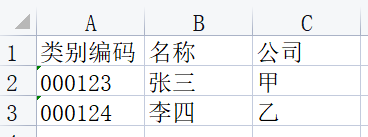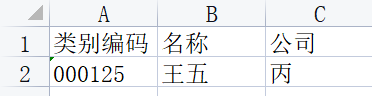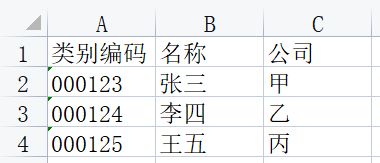• 如何用python合并表格
2021-09-01 23:26:10

# python 合并多个表格

亲测完毕，开森
版本：python 3.7
xlrd需要安装1.2版本

import xlrd,xlsxwriter,glob
import os

biao_tou = "NULL"
wei_zhi = "NULL"

# 获取要合并的所有excel表格
def get_exce():
global wei_zhi
wei_zhi = input("请输入Excel文件所在的目录：")
all_excel = glob.glob(wei_zhi + "*.xlsx")
print("该目录下有" + str(len(all_excel)) + "个excel文件")
if(len(all_excel) == 0):
return 0
else:
for  i in range(len(all_excel)):
print(all_excel[i])
return all_excel

# 打开Excel文件
def open_excel(name):
fh = xlrd.open_workbook(name)
return fh

# 获取Excel文件下所有Sheet
def get_sheet(fh):
sheets = fh.sheets()
return sheets

# 获取sheet下有多少行数据
def get_sheetrow_num(sheet):
return sheet.nrows

# 获取sheet下的数据
def get_sheet_data(sheet,row):
for i in  range(row):
if (i == 0):
global biao_tou
biao_tou = sheet.row_values(i)
continue
values = sheet.row_values(i)
all_data1.append(values)
return  all_data1

if __name__=='__main__':
all_excel = get_exce()
# 得到要合并的所有excel表格数据
if (all_excel == 0):
print("该目录下无.xlsx文件！请检查您输入的目录是否有误！")
os.system('pause')
exit()

all_data1 = []
# 用于保存合并的所有行的数据

# 下面开始文件数据的获取
for excel in  all_excel:
fh = open_excel(excel)
# 打开文件
sheets = get_sheet(fh)
# 获取文件下sheet的数量

for sheet  in  range(len(sheets)):
row = get_sheetrow_num(sheets[sheet])
# 获取一个sheet下的所有数据的行数

all_data2 = get_sheet_data(sheets[sheet],row)
# 获取一个sheet下的所有行的数据

all_data2.insert(0,biao_tou)
# 表头写入

# 开始文件数据的写入
new_excel = wei_zhi + "test.xlsx"
# 新建的excel文件名字

fh1 = xlsxwriter.Workbook(new_excel)
# 新建一个excel表

# 新建一个sheet表

for i in  range(len(all_data2)):
for j in range(len(all_data2[i])):
c = all_data2[i][j]
net_sheet.write(i,j,c)

fh1.close()

print("文件合并成功请查看" + wei_zhi + "目录下的test.xlsx文件！")

os.system('pause')
os.system('pause')

更多相关内容
• python代码，写的合并表格的小内容

## 搜罗出给定路径下的所有文件

• 这里使用了递归遍历
• 还需要注意剔除不是表格的文件
def get_file_path(file_list, path):
try:
if len(file_list) == 0:
# 获取文件夹下是所有文件夹
file_list = os.listdir(path)
if len(file_list) > 0:
for folder in file_list:
if '.' in folder:
str_list = folder.split('.')
if str_list[len(str_list) - 1] in ['xlsx', 'xls']:
# 将表格存入数组
file_path = path + "\\" + folder
file_path_list.append(file_path)
else:
# 深入下一层文件夹
folder_path = path + "\\" + folder
# 获取该文件夹下的所有内容(表格及文件夹)
file_list_one = os.listdir(folder_path)
if len(file_list) > 0:
# 递归调用,筛选出表格
get_file_path(file_list_one, folder_path)
else:
return
except Exception as e:
print("搜罗出现错误,错误原因如下: ", e)
return
print("表格搜罗完成")



## 合并表格内容

• 这里需要将表头不一致的表格进行分类
def merge_excel(path_list, file_path):
if len(path_list) > 0:
df_list = []
title_list = []
try:
for path in path_list:
# 根据表格路径读取表格内容
# 获取表头
titles = df.columns.values
if len(title_list) == 0:
df_son_list = [df]
df_list.append(df_son_list)
title_list.append(titles)
else:
is_same = False
# 遍历表头数组,根据表头对表格进行分类
for index in range(len(title_list)):
title_name_list = title_list[index]
if len(title_name_list) == len(titles):
for i in range(len(title_name_list)):
# 表头不一致
if title_name_list[i] != titles[i]:
# 出现表头不一致的,就跳出循环,继续检查下一个
is_same = False
break
else:
is_same = True
if is_same:
# 将表格数据存入与其表头相同的表格数组
df_list[index].append(df)
break
if not is_same:
# 表头不同的表格, 往末尾添加
df_son_list = [df]
df_list.append(df_son_list)
title_list.append(titles)
except Exception as e:
print("表格分类出错错误,错误原因如下: ", e)
print("错误路径如下: ", path)
try:
if len(df_list) > 0:
print("文件分类完成, 共有{}类, 开始合并: ".format(df_list.__len__()))
for index in range(len(df_list)):
df_list_son = df_list[index]
# 将读取的内容合并
merge_df = pd.concat(df_list_son, axis=0)
# 设置合并后文件的存储路径
new_file_path = file_path + "-合并后\\" + str(index) + "-合并表格.xlsx"
# 生成表格
merge_df.to_excel(new_file_path, index=0)
except Exception as e:
print("表格合并出错,错误原因如下: ", e)
print("大功告成")



## 调用上面的函数

file_path_list = []
# 存放文件的路径,这种是绝对路径,可以自己根据需要修改为任意磁盘的任意位置
# one_path = 'E:\\01-Python\\ManualPractis\\File\\2022-05-24'
# 存放表格的相对路径,一般只能放在项目当中
one_path = '../File/2022-05-24'
get_file_path([], one_path)
merge_excel(file_path_list, one_path)

展开全文开发语言
• 主要为大家详细介绍了Python将多个excel表格合并为一个表格的方法，具有一定的参考价值，感兴趣的小伙伴们可以参考一下
• 用python合并多个excel的数据到一个表格里,Python源码
• 合并多个excel表格，支持界面操作，操作简单，可浏览文件夹及选择表头行数 使用python实现，界面实现采用wxpython，支持不同格式的表格，需安装anaconda3.5及对应头文件，应用程序较大无法上传，如果需要可以联系...
• 将多个子文件夹下的相同名字的excel文件合并成一个excel，可以直接运行。excel见下面链接 https://amaze.blog.csdn.net/article/details/124889377?spm=1001.2014.3001.5502
• 如果需要实现合并单元格，或者按需调整表格样式，就比较麻烦了。 这时，可以试试本文的主角 —— html-table 包，借助它可生成各种样式的 HTML 表格。 接下来，以一个简单的例子演示 html-table 的常用用法： ...
• python 合并excel 表格 单表头 私信提供测试表格和讲解
• 现要将上面两个表格合并如下： 代码： import pandas as pd import os #文件路径 file_dir1 = 'xxx1.xls' file_dir2 = 'xxx2.xls' #构建新的表格名称 new_filename = 'xxx3' new_list = [] dataframe1 = pd.read_...

示例：两个excel表格如下：现要将上面两个表格合并如下：代码：

import pandas as pd
import os

#文件路径
file_dir1 = 'xxx1.xls'
file_dir2 = 'xxx2.xls'
#构建新的表格名称
new_filename = 'xxx3'
new_list = []

#若要保留类型编码列'000123'数据前面的零，使用converters属性
#保存到新列表中
new_list.append(dataframe1)
#保存到新列表中
new_list.append(dataframe2)
#多个DataFrame合并为一个
df = pd.concat(new_list)
#写入到一个新excel表中
df.to_excel(new_filename,index=False)


展开全文开发语言 excel
• python实现excel合并python操作excel的库xlrd和xlwt，这两个库一个可以读一个可以写当然你可以直接openpyxl这个库，支持读写。代码就是个while循环读取每一个条目比较合并。既然是作业，还是自己写一下，对自己是...

python实现excel合并

python操作excel的库xlrd和xlwt，这两个库一个可以读一个可以写

当然你可以直接用openpyxl这个库，支持读写。

代码就是个while循环读取每一个条目比较合并。

既然是作业，还是自己写一下，对自己是有好处的。用上面的库可以完成你的需求，你只需要去看看官网怎么个库，应该就可以写出来了。

我是喜欢用openpyxl，下面附上官网文档：

openpyxl文档

python如何读取多个excel合并到一个excel中

思路

python xlrd包读取excle文件，然后将文件内容存个列表中，再利用xlsxwriter将内容写入到一个新的excel文件中

完整代码

# -*- coding: utf-8 -*-#将多个Excel文件合并成一个import xlrdimport xlsxwriter#打开一个excel文件def open_xls(file):

fh=xlrd.open_workbook(file)    return fh#获取excel中所有的sheet表def getsheet(fh):

return fh.sheets()#获取sheet表的行数def getnrows(fh,sheet):

table=fh.sheets()[sheet]    return table.nrows#读取文件内容并返回行内容def getFilect(file,shnum):

fh=open_xls(file)

table=fh.sheets()[shnum]

num=table.nrows    for row in range(num):

rdata=table.row_values(row)

datavalue.append(rdata)    return datavalue#获取sheet表的个数def getshnum(fh):

x=0

sh=getsheet(fh)    for sheet in sh:

x =1

return xif __name__=='__main__':    #定义要合并的excel文件列表

allxls=['F:/test/excel1.xlsx','F:/test/excel2.xlsx']    #存储所有读取的结果

datavalue=[]    for fl in allxls:

fh=open_xls(fl)

x=getshnum(fh)        for shnum in range(x):

print("正在读取文件：" str(fl) "的第" str(shnum) "个sheet表的内容...")

rvalue=getFilect(fl,shnum)    #定义最终合并后生成的新文件

endfile='F:/test/excel3.xlsx'

wb1=xlsxwriter.Workbook(endfile)    #创建一个sheet工作对象

ws=wb1.add_worksheet()    for a in range(len(rvalue)):        for b in range(len(rvalue[a])):

c=rvalue[a][b]

ws.write(a,b,c)

wb1.close()

print("文件合并完成")1234567891011121314151617181920212223242526272829303132333435363738394041424344454647484950515253545556575859606162

源文件excel1:

源文件excel2:

运行结果：

合并后的excel3：

python处理excel合并表格

通过结果可以发现，valuepack之后，转化为了一段二进制字节而unpack可以把该字节串再转一个元组，但是值得注意的是对于float的精度发生了改变，这是由一些比如操作系统等客观因素所决定的。

打包之后的数据所占用的字节数与C语言中的struct十分相似。

如何用python合并两个excel表格

通过结果可以发现，valuepack之后，转化为了一段二进制字节而unpack可以把该字节串再转一个元组，但是值得注意的是对于float的精度发生了改变，这是由一些比如操作系统等客观因素所决定的。

打包之后的数据所占用的字节数与C语言中的struct十分相似。

用Python导出工程文件两个子页里的数据成为两个exc...

通过结果可以发现，valuepack之后，转化为了一段二进制字节而unpack可以把该字节串再转一个元组，但是值得注意的是对于float的精度发生了改变，这是由一些比如操作系统等客观因素所决定的。

打包之后的数据所占用的字节数与C语言中的struct十分相似。

展开全文• ## Python合并 Excel 表格

千次阅读 2019-12-13 08:18:00
作者：TED 来源：TEDxPY之前曾尝试 Python 写过整理 Excel 表格的代码，记录在《Python 自动整理 Excel 表格》中。当时也是自己初试 p...
• 主要为大家详细介绍了python合并同类型excel表格的方法，具有一定的参考价值，感兴趣的小伙伴们可以参考一下
• 主要介绍了Python基于xlrd模块处理合并单元格,文中通过示例代码介绍的非常详细，对大家的学习或者工作具有一定的参考学习价值,需要的朋友可以参考下
• 主要介绍了Python实现合并excel表格的方法,结合实例形式分析了Python合并Excel表格的原理、实现步骤与相关操作技巧,需要的朋友可以参考下
• 这个pandas的groupby可能实现，代码供你参考# coding: utf-8from __future__ import unicode_literalsimport pandas as pdlst = [{"ID": "1", "订单号": "123456", "用户名": "路人甲", "产品": "XX用品", "颜色":...
• 利用Python，将多个excel文件合并为一个文件 思路 利用python xlrd包读取excle文件，然后将文件内容存入一个列表中，再利用xlsxwriter将内容写入到一个新的excel文件中。 完整代码 # -*- coding: utf-8 -*- #将多...
• 1 场景合并多个word...2 方案采用python实现，使用python中的python-docx和docxcompose结合的方式来实现此功能。优点：跨平台，不依赖office中的word组件Word.Application，可以部署在inux环境中。底层不依赖offi...
• 因为有无数张excel表格合并，就是简单的格式一致的表格，想去掉重复的表头，竖直合并就行。但唯一的要求是保持数据原格式。</strong></p> 尝试了pandas contat，发现合成的完整sheet里...
• 使用Python实现excel表格合并： 一个excel文件的多sheet合并 文件夹下多个Excel文件的合并（单个文件的多sheet也会自动合并） 源码（仅供参考，个需自行修改）： import tkinter as tk from tkinter import ...
• dir = input("请输入待合并文件的路径：\n") #设置工作路径 frames = [] #存放导入的文件 for root, dirs,files in os.walk(dir): #返回一个三元组root是遍历的文件夹， # dirs是root下的所有目录，files是root下的...
• 主要介绍了python合并多个excel文件的示例，帮助大家提高办公效率，感兴趣的朋友可以了解下
• 参考了这个大佬的代码 使用Python合并多个Excel文件 把大佬的代码稍微做了修改。 # lib import os import xlrd import xlsxwriter # 在下方输入需要合并的文件所在文件夹位置 path='C:/Users/11383/Desktop/平均分'...
• 这次给大家带来python怎么批量合并excel表格python批量合并excel表格的注意事项有哪些，下面就是实战案例，一起来看一下。合并单元格合并单元格相信大家都会，比如下面这段简单的代码就可以实现：app='Word'word=...
• 合并表格要注意的是设置表格的表头，也就是是将表格第一列设置为合并表格的索引，这样合并表格的时候就会合并两个表格第一列的元素相同的行。方法一：使用join合并...
• 将一份表格文件中不同 sheet 单页面中的数据汇总整理到一起 python 自动整理 excel表格 摘要：将两份表格通过共有的数据信息进行合并融合给无网络的办公电脑插上 python小翅膀摘要：windows 办公电脑在无网络情况下 ...
• 二、merge “合并” df = pd.merge(left, right, on =["ID", "ID"]) # 实战项目中，同一个企业的数据依据 year 竖向排列，要改为横向合并。 def merge_year(data): temp = pd.merge(data[data['year'] ==2016], ...
• 原标题：Python实战：合并 Excel 表格 首先是需求一：有 表 A.xlsx 和 表 B.xlsx，想将其纵向合并成同一张表格: 以及需求二：想在 表 C.xlsx 中提取第三列、在 表 D.xlsx 中提取前两列，整合成新的表格： 如果不用......